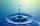Barrel of oil

Barrel of oil weighs 283 kg. When it mold 26% oil, weighed 216 kg. What is the mass of the empty barrel?

Result

m =  25.308 kg

Solution:

m + o = 283
m + (1-26/100)*o = 216

m+o = 283
100m+74o = 21600

m = 32913 ≈ 25.307692
o = 335013 ≈ 257.692308

Calculated by our linear equations calculator.

Leave us a comment of example and its solution (i.e. if it is still somewhat unclear...):Be the first to comment!To solve this example are needed these knowledge from mathematics:

Our percentage calculator will help you quickly calculate various typical tasks with percentages. Do you have a system of equations and looking for calculator system of linear equations? Tip: Our volume units converter will help you with converion of volume units.

Next similar examples:

1. Seeds 2How many seeds germinated from 1000 pcs, when 23% no emergence?
2. Container with waterThe weight of a container with water is 2.48 kg. If the cast 75% water, the container with water has a weight of 0.98 kg. Determine the weight of the empty container. How much water was originally in the container?
3. NumberWhat number is 20 % smaller than the number 198?
4. ClassIn a class are 32 pupils. Of these are 8 boys. What percentage of girls are in the class?
5. GlovesI have a box with two hundred pieces of gloves in total, split into ten parcels of twenty pieces, and I sell three parcels. What percent of the total amount I sold?
6. TVsProduction of television sets increased from 3,500 units to 4,200 units. Calculate the percentage of production increase.
7. IronIron ore contains 57% iron. How much ore is needed to produce 20 tons of iron?
8. PercentsHow many percents is 900 greater than the number 750?
9. EquationsSolve following system of equations: 6(x+7)+4(y-5)=12 2(x+y)-3(-2x+4y)=-44
10. EnrollmentThe enrollment at a local college increased 4% over last year's enrollment of 8548. Find the increase in enrollment (x1) and the current enrollment (x2).
11. TreesAlong the road were planted 250 trees of two types. Cherry for 60 CZK apiece and apple 50 CZK apiece. The entire plantation cost 12,800 CZK. How many was cherries and apples?
12. Two numbersWe have two numbers. Their sum is 140. One-fifth of the first number is equal to half the second number. Determine those unknown numbers.Calculate how many percent will increase the length of an HTML document, if any ASCII character unnecessarily encoded as hexadecimal HTML entity composed of six characters (ampersand, grid #, x, two hex digits and the semicolon). Ie. space as: &#x20;The ball was discounted by 10 percent and then again by 30 percent. How many percent of the original price is now?Theatrical performance was attended by 480 spectators. Women were in the audience 40 more than men and children 60 less than half of adult spectators. How many men, women and children attended a theater performance?If every tenth apple on the tree is rotten it can be expressed by percentages: 10% of the apples on the tree is rotten. Tell percent using the following information: a. in June rained 6 days b, increase worker pay 500 euros to 50 euros c, grabbed 21 froHow many percent is 432 out of 434?